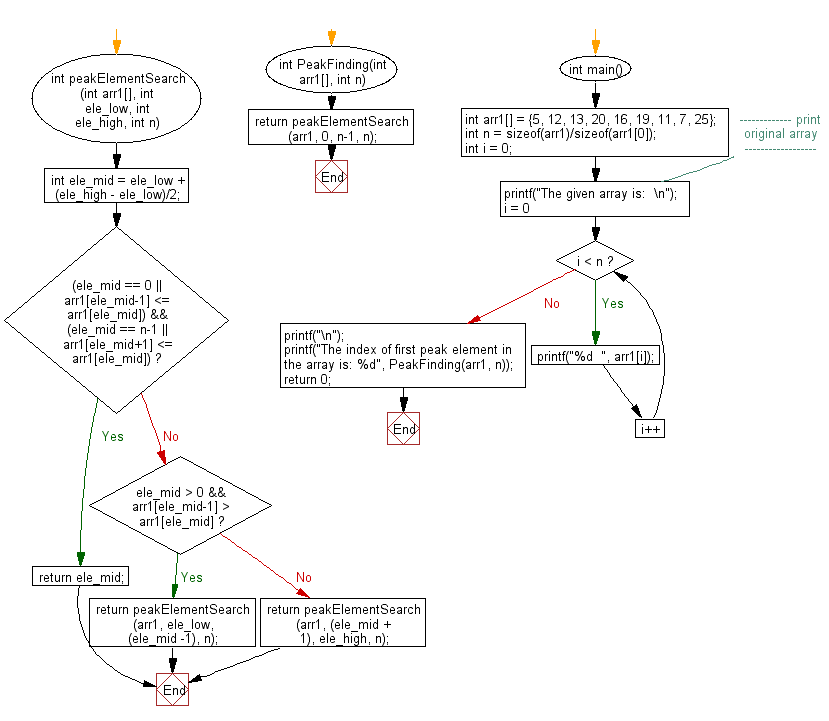﻿ C exercises: Find the index of first peak element in a given array - w3resource# C Exercises: Find the index of first peak element in a given array

## C Array: Exercise-97 with Solution

Write a program in C to find the index of first peak element in a given array.

Sample Solution:

C Code:

``````#include<stdio.h>

int peakElementSearch (int arr1[], int ele_low, int ele_high, int n)
{
int ele_mid = ele_low + (ele_high - ele_low)/2;
if ((ele_mid == 0 || arr1[ele_mid-1] <= arr1[ele_mid]) && (ele_mid == n-1 || arr1[ele_mid+1] <= arr1[ele_mid]))
return ele_mid;
else if (ele_mid > 0 && arr1[ele_mid-1] > arr1[ele_mid])
return peakElementSearch(arr1, ele_low, (ele_mid -1), n);
else return peakElementSearch(arr1, (ele_mid + 1), ele_high, n);
}
int PeakFinding(int arr1[], int n)
{
return peakElementSearch(arr1, 0, n-1, n);
}

int main()
{
int arr1[] = {5, 12, 13, 20, 16, 19, 11, 7, 25};
int n = sizeof(arr1)/sizeof(arr1);
int i = 0;
//------------- print original array ------------------
printf("The given array is:  \n");
for(i = 0; i < n; i++)
{
printf("%d  ", arr1[i]);
}
printf("\n");
//-----------------------------------------------------------
printf("The index of first peak element in the array is: %d", PeakFinding(arr1, n));
return 0;
}
```
```

Sample Output:

```The given array is:
5  12  13  20  16  19  11  7  25
The index of first peak element in the array is: 3
```

Pictorial Presentation:Flowchart:C Programming Code Editor:

Improve this sample solution and post your code through Disqus.

Next: C Pointer Exercises Home

-->
﻿